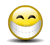Author Topic: Circles and chords - homework question  (Read 1658 times)sparky961Circles and chords - homework question
« on: February 19, 2017, 12:09:06 AM »
I've had the chance to be working out of a math textbook lately and I was hoping that someone could help me understand why I'm not agreeing with the "correct" answer for this question.

See the attached image for the question and diagram.

The answers provided in the back of the book are as follows:

9. a. (1) 41°
(2) 139°
b. (1) 37° 30'
(2) 142° 30'

What I don't get is how angle 1 can be anything other than 36°.  Is the picture somehow misleading me?  Is there a mistake?  This is driving me nuts.

.... Ok, and in the process of preparing everything to put on here, I figured it out.  But I decided to leave it all here because it seems a waste to delete it all.

The second attachment is my screenshot trying to figure it out using SolidWorks.  The picture in the textbook sure looks to me like they're giving a dimension for the angle formed by the intersecting lines.  But the answers only make sense if you interpret this as the angle of the arc between the line endpoints on the circle.

<sigh>

Well, thanks for reading this.... it helped me to figure it out.Pete.Re: Circles and chords - homework question
« Reply #1 on: February 19, 2017, 02:37:31 AM »
Whomever wrote that question must have had a few shandies I think.Lew_Merrick_PE

• Hero Member
•• Posts: 665Re: Circles and chords - homework question
« Reply #2 on: February 19, 2017, 12:26:12 PM »
1) The figure, as presented, is an ellipse rather than a circle.

2) There is (at least 1) piece of "missing information" that allows you to coordinate the angular values.  Otherwise, you have to assume things that may not be true.sparky961Re: Circles and chords - homework question
« Reply #3 on: February 19, 2017, 01:33:49 PM »
1) The figure, as presented, is an ellipse rather than a circle.

2) There is (at least 1) piece of "missing information" that allows you to coordinate the angular values.  Otherwise, you have to assume things that may not be true.

Indeed the question, as written, is a bit lacking.  I didn't consider that the figure would represent an ellipse though.  The unit covers circles, so it is a very safe assumption here, but do you say this because there is no diameter or radius specified; because there is nothing to clearly indicate it is a circle?

For what it's worth, this is from "Mathematics for Machine Technology", Smith & Peterson 6e, page 338 - the "industry standard" textbook on the subject.  Also for what it's worth, it isn't the first mistake or misleading information I've found in the same text.philfRe: Circles and chords - homework question
« Reply #4 on: February 19, 2017, 04:16:11 PM »
Hi Sparky,

It's misleading that that the angle does look as though it's the angle between the two lines and not the angle between the radii between the circle centre and the ends of the lines. As you've already said Ang 1 would be 36 (or B) degrees if it was as the diagram appears to show.)Angles D, F & G are easily found.

D = (180 - A - C)/2 = 90 - A/2 - C/2
F = (180 - A)/2 = 90 - A/2
G = (180 - B - C)/2 = 90 - B/2 - C/2

E = F - D = 90 - A/2 - 90 + A/2 + C/2 = C/2

Ang 1 = 180 - E - F - G = 180 - C/2 - 90 + A/2 -90 + B/2 + C/2

At the end of the day it comes simply down to Ang 1 = A/2 + B/2.

This is true irrespective of angle C as the Cs cancel out.

Phil.
« Last Edit: July 13, 2017, 12:59:20 PM by philf »
Phil Fern
Location: Marple, Cheshiresparky961Re: Circles and chords - homework question
« Reply #5 on: February 19, 2017, 04:36:30 PM »
I was going to ask where you're getting the C's from, but when you later say that they cancel out it results in a good proof of the theorem the book is teaching.